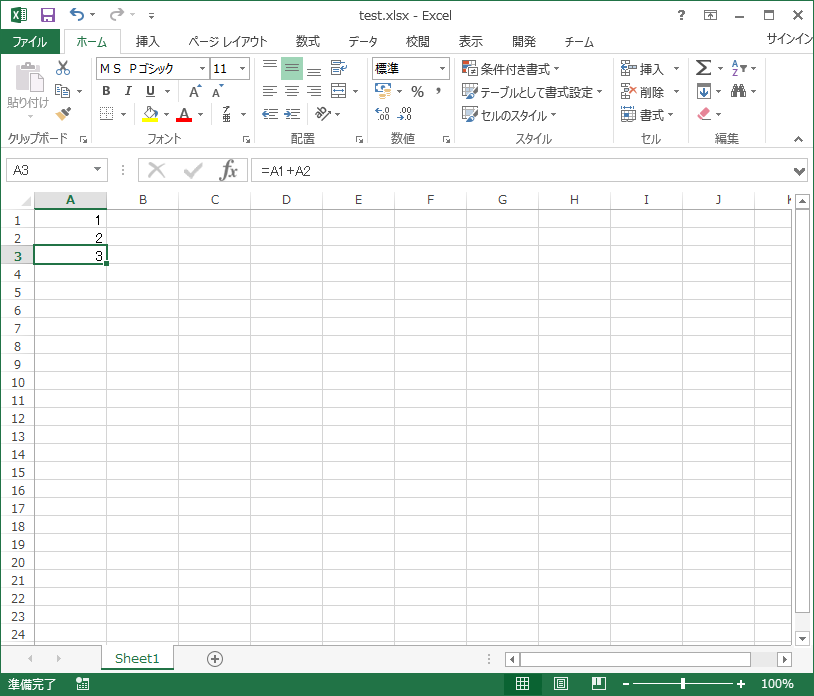# Importing Excel data in Java 3

Again, it's Apache POI, a continuation of the last time. https://qiita.com/Mk-4000/items/5d52b4b92ed5f8bf23f5

# Contents of this time

Last time I wrote how to get each cell type, ** CellType.FORMULA ** Method at the time of getCellFormula() Then, I found that the formula itself in the cell can be obtained as a String type. So what if you want the result of that formula? What to do when you say.

# What to do specifically

I searched for something that I couldn't do in various ways and referred to this article. http://shin-kawara.seesaa.net/article/159878953.html

** Formula Evaluator interface ** There was something called.

This evaluates the formula cell. Make it a value. In short, it helps a lot to get the calculated value.

Also, ** CreationHelper interface ** It seems to use also. This is the object that handles the instantiation of the concrete class. I think it's the one that creates various POI objects.

Prepare these two,

1. Prepare CreationHelper from Workbook object (getCreationHelper ())
2. Create FormulaEvaluator through CreationHelper (createFormulaEvaluator ())
3. Calculate the target cell using FormulaEvaluator.evaluateInCell ()

It is a flow like.

FormulaEvaluator.evaluateInCell(cell) A method that puts a cell in the argument, evaluates and calculates the formula of the cell included in the argument, and returns the result

If you use this, you can get the calculated value even with a mathematical formula.

## Implementation result

#### `SamplePOI3.java`

``````
private static String getCellStringValue(Cell cell) {
String retStr;

CellType cellType = cell.getCellType();
switch (cellType) {
case STRING:
retStr = cell.getStringCellValue();
break;
case NUMERIC:
retStr = String.valueOf(cell.getNumericCellValue());
break;
case BOOLEAN:
retStr = String.valueOf(cell.getBooleanCellValue());
break;
case FORMULA:

//Get function result
Workbook wb = cell.getSheet().getWorkbook();
CreationHelper ch = wb.getCreationHelper();
FormulaEvaluator fe = ch.createFormulaEvaluator();
retStr = String.valueOf(getCellStringValue(fe.evaluateInCell(cell)));

break;
case ERROR:
retStr = String.valueOf(cell.getErrorCellValue());
break;
default:
retStr = "";
break;
}
return retStr;
}
``````#### `samplePOI3.result`

``````
1.0
2.0
3.0
``````